Mobile QR Code1. (Division of Electronic Engineering, Jeonbuk National University, Jollabuk-do 561-756, Korea.)
2. (Samsung Electronics System LSI, 1-1, Samsungjeonja-ro, Hwaseong-si, Gyeonggi-do 474-1, Korea. )

CMOS, complementary source follower, concurrent, dual-band, feedback, minimum noise figure, noise figure, noise matching, optimum noise impedance, WLAN

## I. INTRODUCTION

The most recent and interesting evolution of the modern RF transceiver is toward a low-cost single-chip CMOS radio supporting multiband (MB), multimode (MM), and multi-standard (MS). With the explosive growth of WiFi, which is considered to be the most promising wireless technology for the Internet of Things (IoT), the wireless local area network (WLAN) system also requires a highly integrated CMOS transceiver supporting MB/MM with low cost. Many studies have reported single-chip 2.4/5.2 GHz dual-band CMOS transceivers for IEEE 802.11a/b/g WLAN applications (1-3). Recently, the demand for higher data-rates for the broadband WLAN system has driven a new standard of IEEE 802.11ax to adopt methods to increase the channel bandwidth by concurrent reception and carrier aggregation, and it brings new technical challenges for transceiver design because more signals in intra-bands or inter-bands will be processed simultaneously (4).

Contrast to the conventional receiver architectures where simultaneous reception at two different frequencies can only be performed by building two independent signal paths, the concurrent 2.4/5.2 GHz dual-band receiver architecture of Fig. 1 including this work employs a non-continuous carrier aggregation technique reported in the long term evolution (LTE) receiver of (5), and as a result, enables simultaneous operation at two different frequencies without dissipating twice as much power or a significant increase in hardware complexity. However, because the double quadrature image rejection mixer stage for the carrier aggregation causes a higher noise floor in comparison with the conventional low-IF/zero-IF receiver, a low noise LNA with sufficiently high gain at two frequency bands is required for high sensitivity performance. In addition, it is better to provide a good out-of-band (OOB) rejection characteristic in order to handle strong interferes.

In this paper, a concurrent dual-band CMOS partial feedback LNA is designed for advanced WLAN applications supporting concurrent reception and carrier aggregation. This is the first suggestion of concurrent dual-band LNA optimizing noise and input reflection coefficient ($\textit{S}$$_{11}) at both 2.4 and 5.2 GHz frequency bands through an input noise matching network and a partial feedback. Section II describes a circuit design of the proposed concurrent dual-band LNA, including an analytical study on its optimum noise impedance (\textit{Z}$$_{opt}$), minimum noise figure ($\textit{NF}$$_{min}), and concurrent input noise matching network. In addition, a partial feedback-based noise and \textit{S}$$_{11}$ optimization technique at both 2.4 and 5.2 GHz frequency bands is presented. Section III reports the simulation results of the proposed LNA, followed by the conclusion in Section IV.

Fig. 1. An architecture for concurrent 2.4/5.2 GHz dual-band receiver employing non-continuous carrier aggregation technique reported in the long term evolution (LTE) receiver of (5).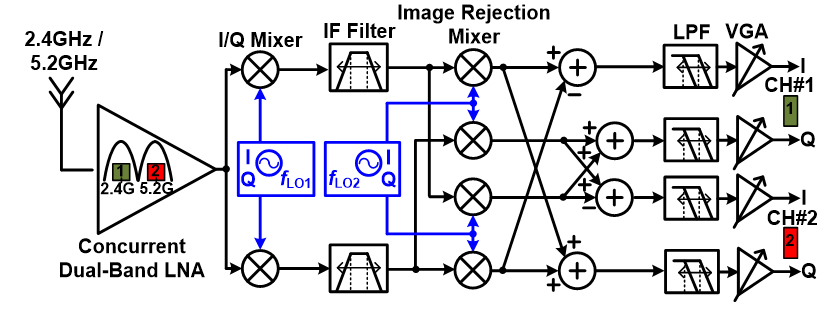## II. DESIGN OF 2.4/5.2 GHZ CONCURRENT DUAL-BAND LNA

There are several LNA design approaches for concurrent reception and carrier aggregation applications, including parallel, concurrent, or wideband LNAs. Parallel LNAs are implemented by placing several individual narrowband LNAs for each band. The out-of-band attenuation characteristic from the input matching network relaxes the linearity burden of the RF front-end, but the complexity and occupied silicon area grow rapidly as the number of frequency bands increases. For the wideband LNA, many standards are supported in a wide frequency range with low complexity and small silicon area. However, its noise performance is much poorer than that in parallel LNAs and the higher linearity is required due to no OOB rejection characteristic.

The concurrent LNA is a compromise solution between parallel LNAs and wideband LNA. Many studies have reported concurrent multi-band LNAs in CMOS (6-9). Most of them adopted an inductively degenerated common-source (CS) topology with concurrent dual-band input matching networks. Unfortunately, all of them consumed a large silicon area due to many on-chip spiral inductors besides an external input matching network and suffered from higher noise figure caused by on-chip source degeneration inductor at higher frequencies. In addition, it is almost impossible to support wideband operation through the reconfiguration of LNA core and board components for customer-specific applications.

Fig. 2 shows the schematic of the proposed concurrent dual-band LNA. The inverter-based input transconductance stage ($\textit{M}_{1}$$_{n} and \textit{M}_{1}$$_{p}$) directly drives two parallel cascode transistors ($\textit{M}_{2}$$_{n}) with 2.4 and 5.2 GHz \textit{LC} loads, and the output signals splitting into two resonators are combined through a complementary source follower 2 (CSF2, \textit{M}_{4}$$_{n}$ and $\textit{M}$$_{4}$$_{p}$). At this figure, the CSF1 ($\textit{M}$$_{3}$$_{n}$ and $\textit{M}$$_{3}$$_{p}$) for partial feedback at only 2.4 GHz becomes disabled. The complementary circuit configuration enhances the effective transconductance of the input stage and output buffer by current reuse, and provides more linear feedback operation with low second-order and third-order harmonic distortion in designing a voltage-mode feedback buffer amplifier for the feedback operation (10). The cascode transistors isolate two $\textit{LC}$ resonators and greatly reduce the Miller capacitance of the input stage.

Because the LNA determines the NF and $\textit{S}$$_{11} performance of the whole receiver, the simultaneous noise and input impedance matching (SNIM) technique is highly required for the LNA. It is well known that SNIM is a condition that the input impedance (\textit{Z}$$_{in}$) and the conjugated to $\textit{Z}$$_{opt} (\textit{Z}$$_{opt}$$^{*}) of the amplifier are simultaneously matched to the source impedance through the matching network (11). Therefore, firstly, it is important to analyze \textit{Z}$$_{opt}$, $\textit{NF}$$_{min}, and \textit{Z}$$_{in}$ of the proposed LNA of Fig. 2 at both 2.4 and 5.2 GHz frequency bands for the SNIM.

### 1. Analysis of Optimum Noise Impedance (Zopt) and Minimum Noise Figure (NFmin), and Input Impedance (Zin)

Fig. 3 shows the simplified schematic for calculation of $\textit{Z}$$_{opt} and \textit{NF}$$_{min}$ at 2.4 and 5.2 GHz frequency bands. Because the noise contribution from the CSF output buffer is small, the dominant noise sources of $\textit{V}$$_{n,Rg}, \textit{I}$$_{n,}$$_{1}$$_{n}$, $\textit{I}$$_{n,}$$_{1}$$_{p}, and \textit{I}$$_{n,}$$_{2}$$_{n}$ are only considered for the calculation. $\textit{V}$$_{n,Rg} denotes the noise voltage from the distributed gate resistance (\textit{R}$$_{g}$) of $\textit{M}$$_{1}$$_{n}$ and $\textit{M}$$_{1}$$_{p}$, and it is given by

##### (1)
$\overline{V_{n, R g}^{2}}=4 k T R_{g}$, where $R_{g} \approx \frac{R_{g_{g} h} W_{f}}{3 L_{f} N_{f}}$

Fig. 2. Schematic of the proposed concurrent dual-band LNA. At this figure, the complementary source follower 1 (CSF1) for partial feedback at only 2.4 GHz is disabled. $\textit{R}$$_{B} (= 100 kΩ) and \textit{C}$$_{B}$ (= 5 pF) denote the high-value resistor for dc bias injection and ac-coupling capacitor, respectively. The channel length of all MOSFETs is 65 nm.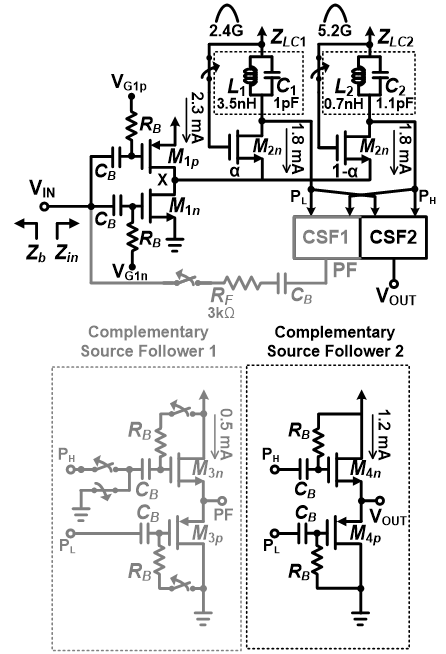Fig. 3. Simplified schematic for calculation of $\textit{Z}$$_{opt} and \textit{NF}$$_{min}$ at 2.4 and 5.2 GHz frequency bands. The dominant noise sources of $\textit{V}$$_{n,Rg}, \textit{I}$$_{n,}$$_{1}$$_{n}$, $\textit{I}$$_{n,}$$_{1}$$_{p}, and \textit{I}$$_{n,}$$_{2}$$_{n}$ are only considered for the calculation. $\textit{V}$$_{n,Rg} denotes the noise voltage from the distributed gate resistance (\textit{R}$$_{g}$) of $\textit{M}$$_{1}$$_{n}$ and $\textit{M}$$_{1}$$_{p}$, and $\textit{I}$$_{n,}$$_{1}$$_{n}, \textit{I}$$_{n,}$$_{1}$$_{p}$, and $\textit{I}$$_{n,}$$_{2}$$_{n} are the channel thermal noise current by \textit{M}$$_{1}$$_{n}, \textit{M}$$_{1}$$_{p}, and \textit{M}$$_{2}$$_{n}, respectively.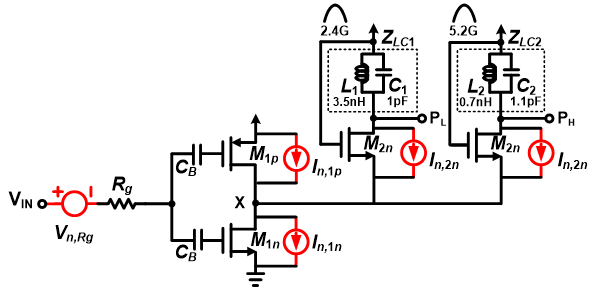where \textit{R}$$_{gsh}$ is the sheet resistance of poly gate and $\textit{W}$$_{f}, \textit{L}$$_{f}$, and $\textit{N}$$_{f} denote the finger width, channel length, and number of fingers of the MOSFET, respectively (12). From (12), \textit{Z}$$_{opt}$ and $\textit{NF}$$_{min} of the two-port network in terms of its noise correlation matrix entries \textit{C}$$_{11}$, $\textit{C}$$_{12}, \textit{C}$$_{21}$, and $\textit{C}$$_{22} are calculated as ##### (2) \frac{1}{Z_{o x t}}=G_{o p x}+j B_{o x t}=\left[\frac{C_{22}}{C_{11}}-\left(\frac{\operatorname{Im}\left(C_{12}\right)}{C_{11}}\right)^{2}\right]^{\frac{1}{2}}+j \frac{\operatorname{Im}\left(C_{12}\right)}{C_{11}} ##### (3) N F_{\min }=1+2\left[\operatorname{Re}\left(C_{12}\right)+\left(C_{11} \cdot G_{\text {opt }}\right)\right] where the matrix entries of the proposed LNA are given by (4), (5), and (6) as functions of channel thermal noise current (\textit{I}$$_{n,}$$_{1}$$_{n}$, $\textit{I}$$_{n,}$$_{1}$$_{p}, and \textit{I}$$_{n,}$$_{2}$$_{n}$), distributed gate resistance ($\textit{R}$$_{g}), and \textit{Y}-parameters of the LNA of Fig. 2 in case of ignoring the induced gate noise of MOSFET. ##### (4) C_{11}=R_{n}=R_{g}+\frac{\overline{I_{n, 1 n}^{2}}+\overline{I_{n, 1 p}^{2}}+\overline{I_{n, 2 n}^{2}}+\overline{I_{n, 2 n}^{2}}}{4 k T\left|Y_{21}\right|^{2}} ##### (5) C_{21}=C_{12}^{*}=Y_{11} \cdot\left(R_{n}-R_{g}\right) ##### (6) C_{22}=\left|Y_{11}\right|^{2} \cdot\left(R_{n}-R_{g}\right) Fig. 4 shows the simulated \textit{Z}$$_{opt}$, $\textit{NF}$$_{min}, and noise circle of the proposed LNA at 2.4 and 5.2 GHz frequency bands on a smith chart. The simulated noise circle was represented by a gradient color scheme. The simulated \textit{NF}$$_{min}$ at 2.4 and 5.2 GHz are 0.8 and 1.6 dB, respectively, and the NF of the LNA increases by 0.25 dB than $\textit{NF}$$_{min} at the outermost noise circle shown in Fig. 4. The calculated \textit{Z}$$_{opt }$was marked with a red star for the comparison with the simulation one marked with a blue star. From (2) and (3), $\textit{Z}$$_{opt} and \textit{NF}$$_{min}$ of the LNA are calculated as 50+j156 Ω and 0.52 dB at 2.4 GHz and 26.5+j52 Ω and 1.55 dB at 5.2 GHz, respectively. The calculated results are well matched to the simulated $\textit{Z}$$_{opt} and \textit{NF}$$_{min}$ at both frequency bands.

Fig. 4. Simulated $\textit{Z}$$_{opt}, \textit{NF}$$_{min}$, and noise circle (NC) of the proposed LNA at 2.4 and 5.2 GHz frequency bands on a smith chart. The simulated noise circle was represented by a gradient color scheme. The calculated $\textit{Z}$$_{opt }was marked with a red star for the comparison with the simulation one marked with a blue star.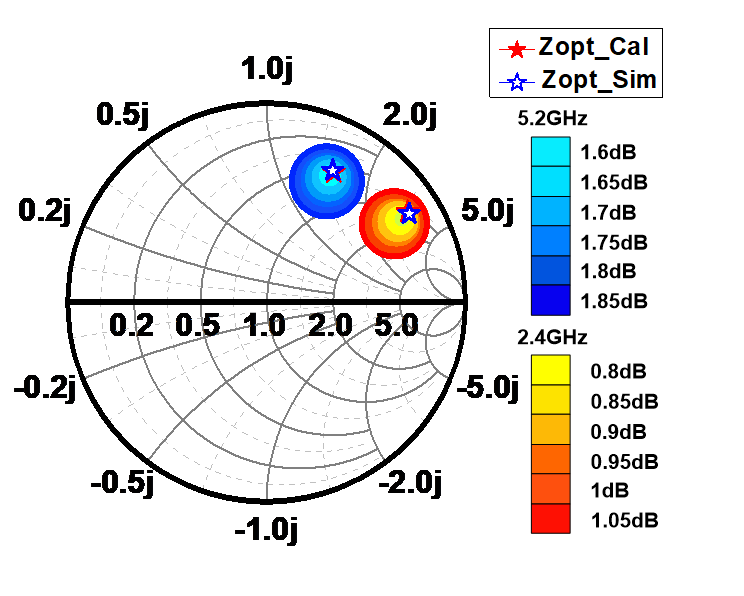Fig. 5. (a) Calculated and simulated \textit{Z}$$_{opt}$ and complex conjugate of $\textit{Z}$$_{in} of the proposed LNA at 2.4 and 5.2 GHz frequency bands and the impedance trajectory of \textit{Z}$$_{b}$ which is the impedance looked back to the LNA through the input matching network, (b) designed concurrent dual-band input matching network.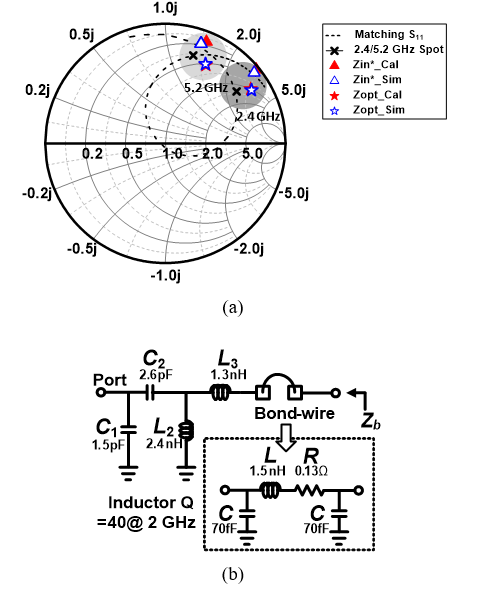### 2. Design of 2.4/5.2 GHz Concurrent Dual-band LNA

It is well known that the cascode inductively degenerated CS LNA employing an additional gate-to-source capacitor can match $\textit{Z}$$_{in}$$^{*}$ with $\textit{Z}$$_{opt}, and as a result, provide the SNIM without the degradation of \textit{NF}$$_{min}$ at a given single operating frequency for all power dissipation levels. However, unfortunately, it is very difficult to obtain SNIM at concurrent dual-band operating frequencies, and its NF increases abruptly at the frequencies away from $\textit{Z}$$_{opt} because a large additional gate-to-source capacitor severely decreases the effective cutoff frequency of the input transistor (11). In Fig. 5, the calculated and simulated complex conjugate of \textit{Z}$$_{in}$ of the proposed LNA at 2.4 and 5.2 GHz are marked with triangle symbol. The proposed LNA shows the inherent mismatch between $\textit{Z}$$_{in}$$^{*}$ with $\textit{Z}$$_{opt}, and it typically requires compromise between the noise and input impedance matching performance. Considering the mismatch in \textit{Z}$$_{opt}$ directly degrades the NF of the LNA and is considered more severe than that in $\textit{Z}$$_{in}$$^{*}$ because some amounts of the mismatch in $\textit{Z}$$_{in}$$^{*}$ are allowed on the LNA performance, it is desirable to make $\textit{Z}$$_{b}, which is the impedance looked back to the LNA through the input matching network, have an impedance trajectory as close as possible to \textit{Z}$$_{opt}$ at 2.4 and 5.2 GHz frequencies while achieving $\textit{S}$$_{11} of less than -10 dB. Fig. 5 shows the designed concurrent dual-band input impedance matching network and simulated impedance trajectory of \textit{Z}$$_{b}$. The symbol ‘X’ indicates the impedance point of $\textit{Z}$$_{b} at 2.4 and 5.2 GHz frequencies. In the simulation, the lumped-element spice model for on-board components and bond-wire was used to consider the finite quality factor (\textit{Q}-factor) and some parasitic elements. As shown in Fig. 5(a) and 6, the impedance trajectory of \textit{Z}$$_{b}$ passes through the midpoint between $\textit{Z}$$_{in}$$^{*}$ with $\textit{Z}$$_{opt} at 5.2 GHz frequency and as a result the LNA can achieve low NF of 2 dB (around \textit{NF}$$_{min}$ of 1.6 dB) with $\textit{S}$$_{11} of less than -10 dB. It is the best way to make an impedance trajectory of \textit{Z}$$_{b}$ pass through the midpoint between $\textit{Z}$$_{in}$$^{*}$ with $\textit{Z}$$_{opt} at 2.4 GHz frequency using an identical input matching network, but it requires a large number of on-board components in designing the input matching network. Based on the same input matching network of Fig. 5(b), the LNA achieves low NF of 1.2 dB (around \textit{NF}$$_{min}$ of 0.8 dB) at 2.4 GHz frequency. However, the $\textit{S}$$_{11} performance of the LNA is very poor. The discrepancy between NF and \textit{NF}$$_{min}$ at both frequencies comes from finite $\textit{Q}$-factor of on-board components and bond-wire.

Fig. 6. Simulated $\textit{NF}$$_{min}, NF, and \textit{S}$$_{11}$ of the proposed LNA with concurrent dual-band input matching network of Fig. 5(b).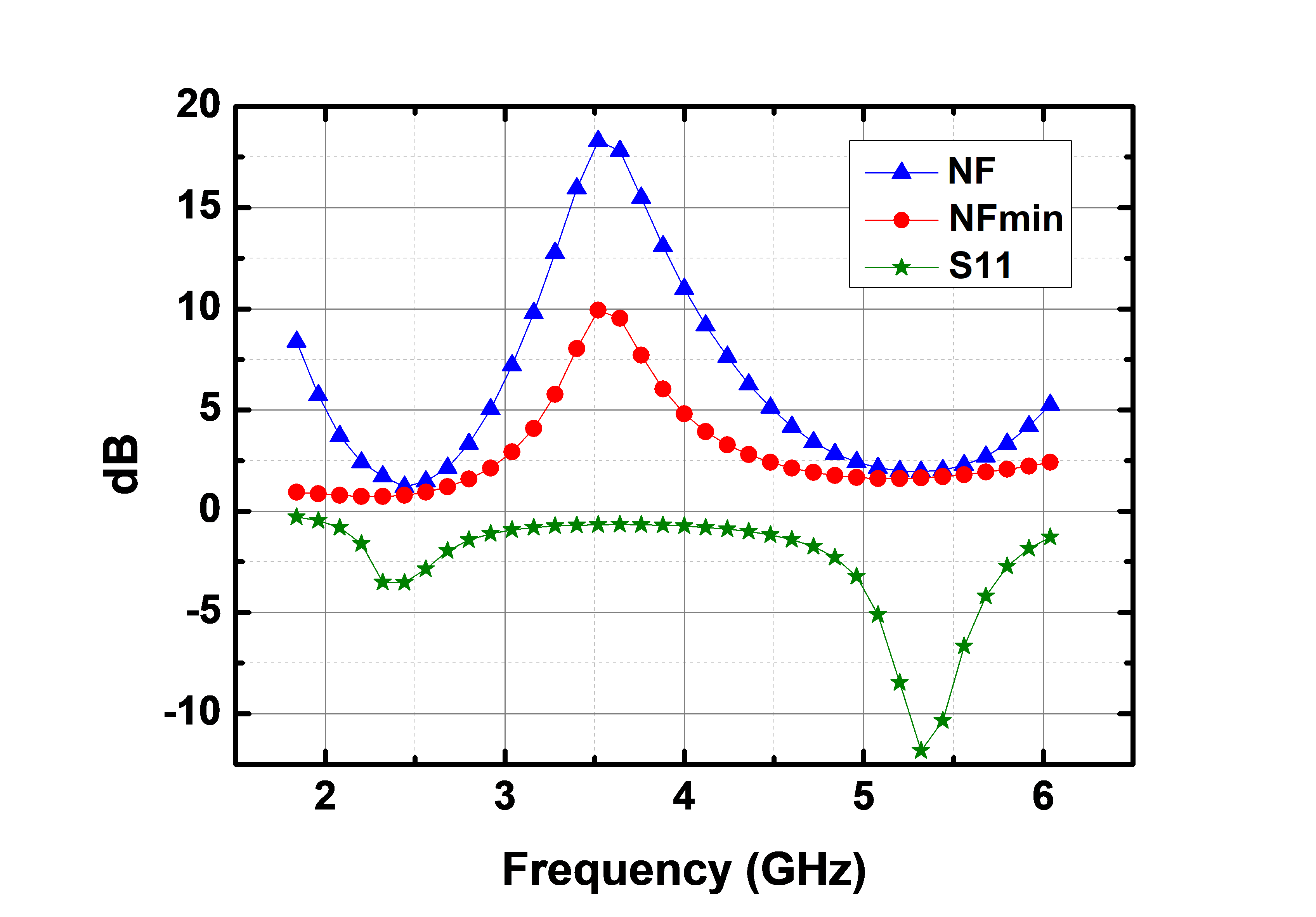Fig. 7. Schematic of the proposed concurrent dual-band partial feedback LNA. At this figure, the complementary source follower 1 (CSF1) for partial feedback at only 2.4 GHz is enabled. The 2.4 GHz feedback path is marked with blue line.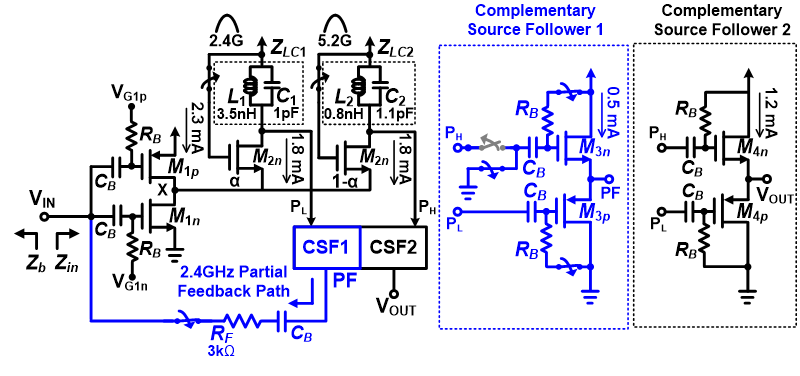Fig. 8. Simulated $\textit{Z}$$_{opt} and \textit{Z}$$_{in}$$^{\mathrm{*}} of the LNA at 2.4 and 5.2 GHz frequency bands with and without partial feedback and the impedance trajectory of \textit{Z}$$_{b}$ through the matching network of Fig. 5(b). PFB means a partial feedback.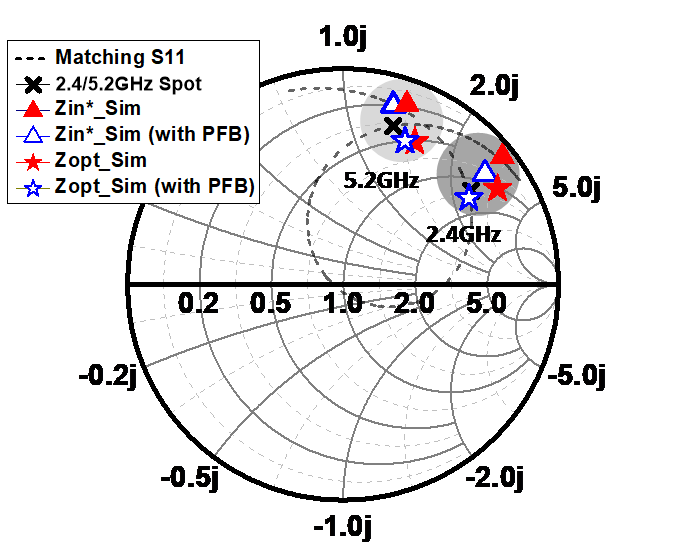Fig. 9. Comparison of $\textit{NF}$$_{min} of the LNA with and without partial feedback. PFB means a partial feedback.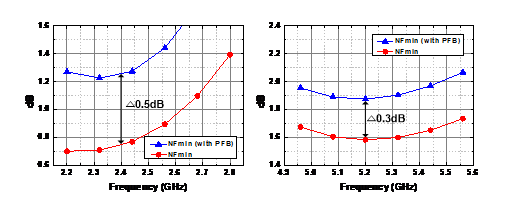### 3. Noise and Input Impedance Matching Optimization Utilizing Partial Feedback Fig. 7 shows the proposed concurrent dual-band LNA employing partial feedback to improve poor \textit{S}$$_{11}$ performance at 2.4 GHz. Compared to the LNA of Fig. 2, the CSF1 for partial feedback at only 2.4 GHz is enabled by closing the switches for dc bias injection. Eventually, the output of 2.4 GHz \textit{LC} resonator becomes connected to the input of the LNA through CSF1 and feedback resistor $\textit{R}$$_{F}. As shown in Fig. 8, the partial feedback increases real part of \textit{Z}$$_{in}$, shifts $\textit{Z}$$_{in}$$^{*}$ with $\textit{Z}$$_{opt} of the LNA, and makes the impedance trajectory of \textit{Z}$$_{b}$ pass through the midpoint between them at 2.4 GHz frequency, while it causes negligible effects on 5.2 GHz reception. One major drawback is the degradation of $\textit{NF}$$_{min} at 2.4 GHz caused by the thermal noise generated from \textit{R}$$_{F}$, but this will not be an issue in terms of dual-band receiver performance because $\textit{NF}$$_{min }at 2.4 GHz is much lower than \textit{NF}$$_{min }$at 5.2 GHz and this allows the LNA some degradation of NF at 2.4 GHz frequency. As shown in Fig. 9, the partial feedback degrades $\textit{NF}$$_{min} of the LNA by 0.5 dB at 2.4 GHz and by 0.3 dB at 5.2 GHz, respectively. ## III. Simulation Results Fig. 10. Simulated \textit{NF}$$_{min}$, NF, forward gain ($\textit{S}$$_{21}), and \textit{S}$$_{11}$ of the proposed concurrent dual-band LNA employing partial feedback and concurrent dual-band input matching network of Fig. 5(b).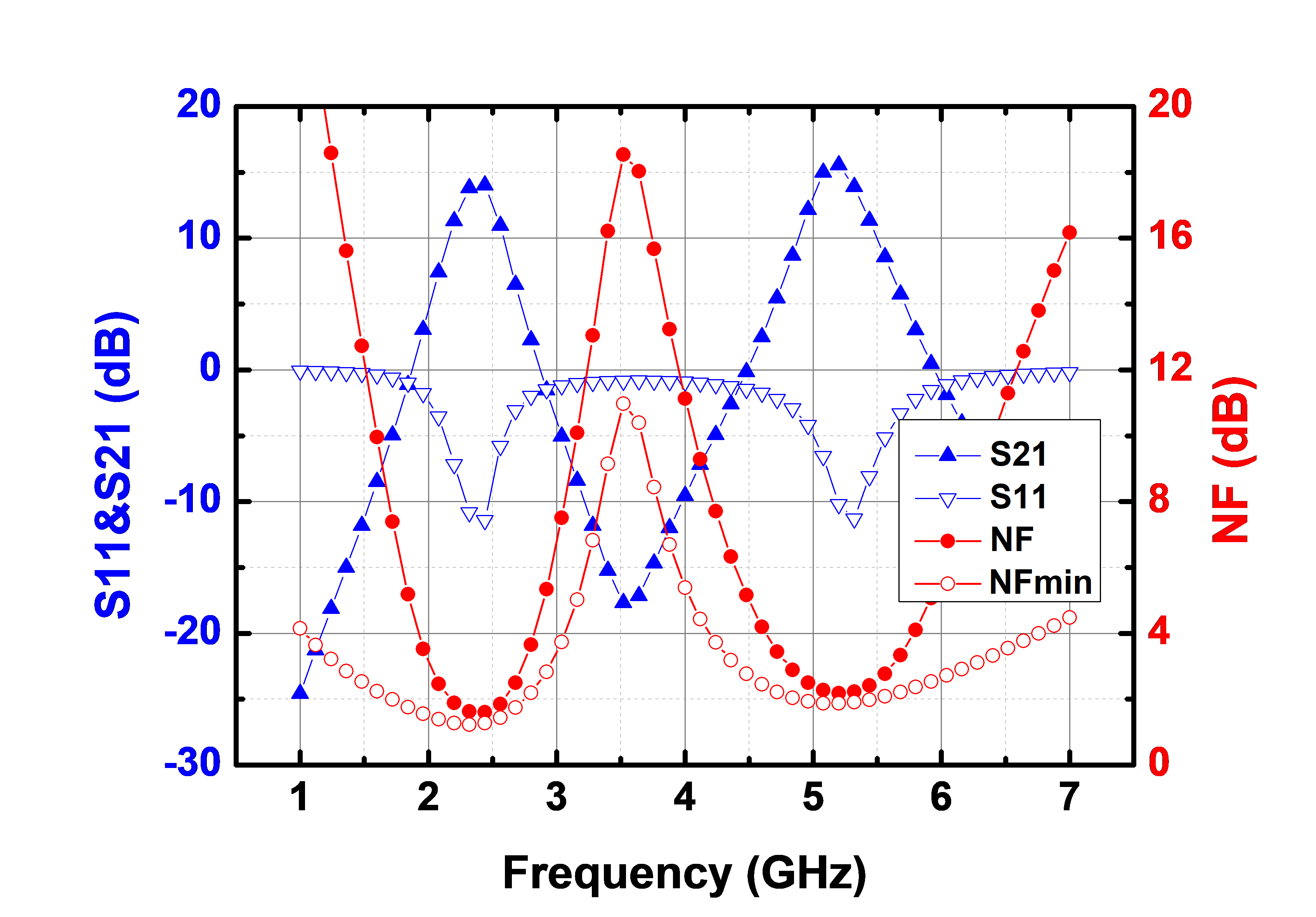Fig. 11. $\textit{NF}$$_{min}, NF, and \textit{S}$$_{11}$ of the proposed concurrent dual-band LNA with and without the partial feedback. The blank and solid symbols denote the simulation results with and without the partial feedback respectively.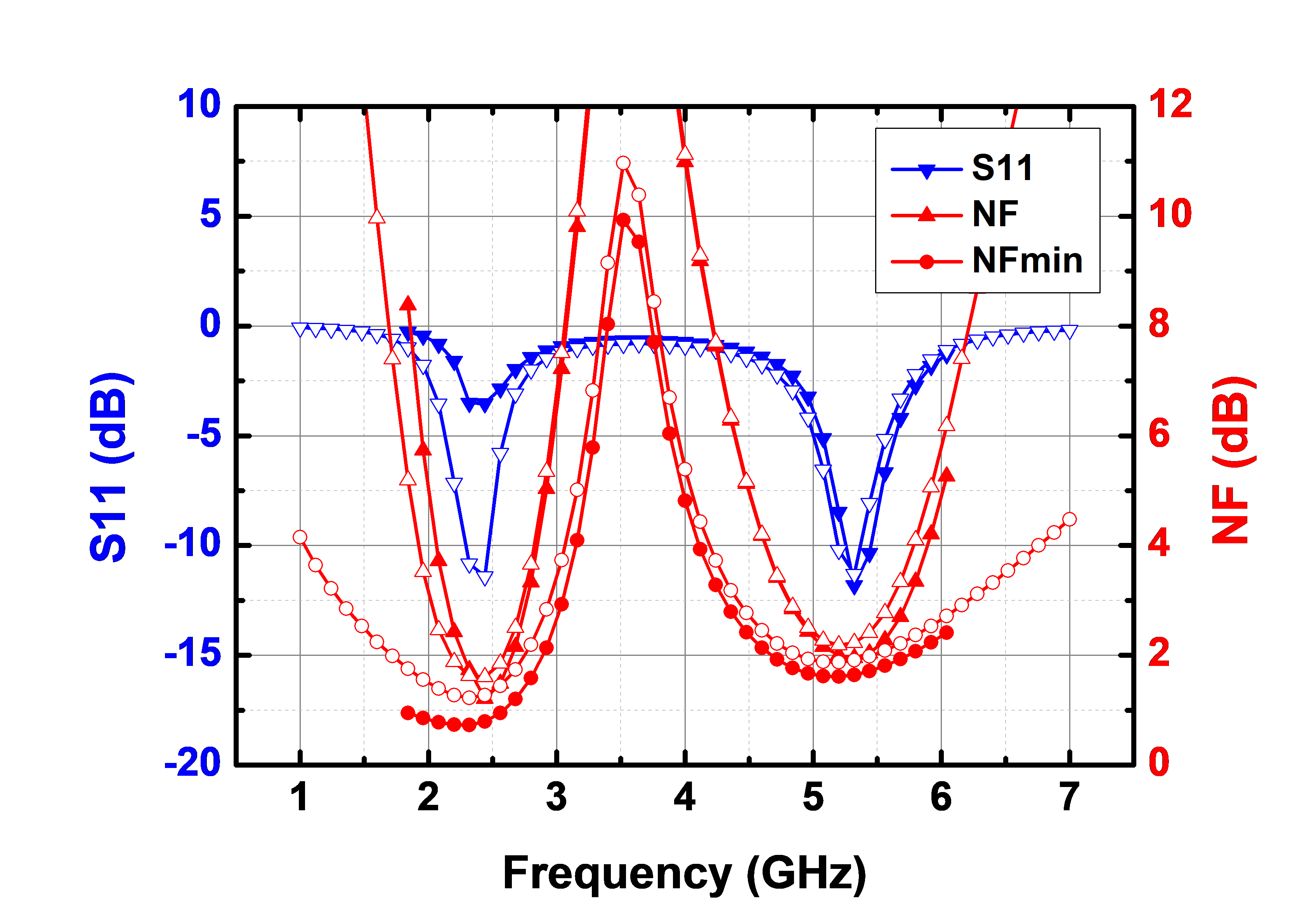The proposed LNA was designed using a 65-nm CMOS technology. It consumes a total current of 6.4 mA (excluding output buffer of CSF2) from a 1.2 V supply voltage. Fig. 10 shows the simulated $\textit{NF}$$_{min}, NF, forward gain (\textit{S}$$_{21}$), and $\textit{S}$$_{11} of the complete concurrent dual-band LNA employing partial feedback and concurrent dual-band input matching network of Fig. 5(b). As predicted, \textit{S}$$_{11}$ of less than -10 dB is achieved at both 2.4 and 5.2 GHz frequencies. The simulated NF of the complete LNA at 2.4 and 5.2 GHz frequencies are 1.6 and 2.2 dB, respectively, and both of them are near around $\textit{NF}$$_{min}. In case of \textit{S}$$_{21}$, the LNA shows a forward gain of greater than 14 dB at both frequencies.

Fig. 11 presents the simulated $\textit{NF}$$_{min}, NF, and \textit{S}$$_{11}$ of the proposed concurrent dual-band LNA with and without the partial feedback. The input impedance matching at dual frequency bands can be achieved through the partial feedback with nearly the same NF.

Fig. 12. Simulated third-order input-referred intercept point (IIP3) of the complete LNA at (a) 2.4 GHz, (b) 5.2 GHz.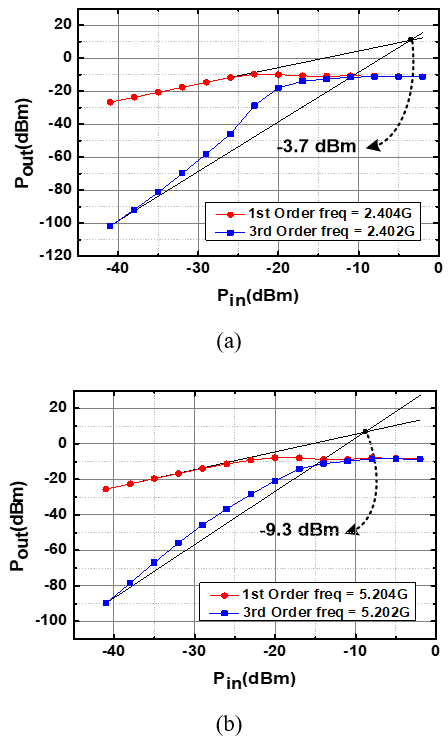Fig. 12(a) and (b) show the simulated third-order input-referred intercept point (IIP3) of the complete LNA at 2.4 and 5.2 GHz frequencies. Two-tone test was performed at both frequency bands in the simulation, and the tone spacing was 2 MHz. The simulated IIP3 of the complete LNA at 2.4 and 5.2 GHz frequencies are -3.7 and -9.3 dBm, respectively.

Table 1 summarizes and compares the performance of the proposed concurrent dual-band LNA against other previous works. Due to a partial feedback-based noise and $\textit{S}$$_{11} optimization technique at both 2.4 and 5.2 GHz frequencies, the proposed concurrent dual-band LNA shows lower NF near around \textit{NF}$$_{min}$ compared to other previous works while showing comparable linearity and power dissipation. To compare the performances of the LNAs, the figure-of-merit (FoM) is defined by (13)

##### (7)
$\mathrm{FoM}=\frac{\text { Freq }[\mathrm{GHz}] \cdot \text { Gain }[\mathrm{abs}] \cdot \mathrm{IIP} 3[\mathrm{~mW}]}{(\mathrm{NF}[\mathrm{abs}]-1) \cdot \mathrm{P}_{\mathrm{DC}}[\mathrm{mW}]}$

## IV. CONCLUSIONS

The concurrent dual-band CMOS LNA optimizing noise and $\textit{S}$$_{11} at both 2.4 and 5.2 GHz frequencies through an input noise matching network and a partial feedback was firstly demonstrated through the simulation for advanced WLAN applications. The proposed LNA achieved low NF (near around \textit{NF}$$_{min}$) with sufficiently high gain and good input impedance matching performance at both frequencies while supporting various modes of narrowband, concurrent dual-band, and wideband easily through hardware reconfigurability.

Table 1. Performance Summary and Comparison

 * ** ** * *This Work Frequency (GHz) 2.4 / 5.2 2.45 / 6 2.4 / 5.2 2.4 / 5.2 2.4 / 5.2 S21 (dB) 21.0 / 12.5 9.4 / 18.9 12.9 / 8.2 19.3 / 17.5 14.0 / 15.5 NF (dB) 2.5 / 2.4 2.8 / 3.8 3.7 / 3.7 3.2 / 3.3 1.6 / 2.2 S11 (dB) -12.7 / -17.4 -12.6 / -21.0 -13.1 / -10.5 -16.8 / -19.4 -11.2 / -10.3 IIP3 (dBm) N/A -4.3 / -5.6 -4.0 / -1.0 -20.1 / -18.1 -3.7 / -9.3 Power Consumption (mW) 10 @ 1.8 V 2.8 @ 1.2 V 7.6 @ 1.0 V 2.4 @ 1.2 V 7.7 @ 1.2 V Process Technology (CMOS) 180-nm 130-nm 180-nm 130-nm 65-nm FoM N/A 3.1 / 32.8 1.8 / 2.7 0.8 / 1.7 7.5 / 4.3

* Simulation results, ** Measurement results

### ACKNOWLEDGMENTS

This paper was supported by research funds of Jeonbuk National University in 2021. This work was also supported by the National Research Foundation of Korea (NRF) grant funded by the Korea government(MSIT)(No. 2021R1A4A1032234), Korea and by the Institute of Information and Communications Technology Promotion (IITP) grant funded by the Korea government (MSIT) under Grant 2018-0-01461. The circuit simulation and EDA tool were supported by the IC Design Education Center (IDEC), Korea.

### REFERENCES

1
Zargari M., Terrovitis M., Jen S. H. -M., Kaczynski B. J., MeeLan Lee , Mack M. P., Mehta S. S., Mendis S., Onodera K., Samavati H., Si W. W., Singh K., Tabatabaei A., Weber D., Su D. K., Wooley B. A., Dec 2004, A single-chip dual-band tri-mode CMOS transceiver for IEEE 802.11a/b/g wireless LAN, IEEE J. Solid-State Circuits, Vol. 39, No. 12, pp. 2239-22492
Maeda T., Matsuno N., Hori S., Yamase T., Tokairin T., Yanagisawa K., Yano H., Walkington R., Numata K., Yoshida N., Takahashi Y., Hida H., Nov 2006, A low-power dual-band triple-mode WLAN CMOS transceiver, IEEE J. Solid-State Circuits, Vol. 41, No. 11, pp. 2481-24903
Gross S., Maimon T., Cossoy F., Ruberto M., Normatov G., Rivkind A., Telzhensky N., Banin R., Ashckenazi O., Ben-Bassat A., Zaguri S., Hara G., Zajac M., shahar N., Shahaf S., Yousef H., Mor E., Eliat Y., Nazimov A., Beer Z., Fridman A., Degani O., Anaheim, Dual-band CMOS transceiver with highly integrated front-end for 450Mb/s 802.11 n systems, in Proc. IEEE RFIC4
Yi X., Yang K., Liang Z., Liu B., Devrishi K., Boon C.C., Li C., Feng G., Regev D., Shilo S., Meng F., Liu H., Sun J., Hu G., Miao Y., San Francisco, A 65nm CMOS carrier-aggregation transceiver for IEEE 802.11 WLAN applications, in Proc. IEEE RFIC5
Hwu S., Razavi B., April 2006, An RF receiver for intra-band carrier aggregation, IEEE J. Solid-State Circuits, Vol. 50, No. 4, pp. 946-9616
Kargaran E., Madadi B., June. 2010, Design of a novel dual-band concurrent CMOS LNA with current reuse topology, in Proc. IEEE ICNIT, Manila, Philippines, pp. 386-3887
Neihart N., Brown J., Yu X., Aug. 2012, A dual-band 2.45/6 GHz CMOS LNA utilizing a dual-resonant transformer-based matching network, IEEE Trans. Circuits Syst. I, Reg. Papers, Vol. 59, No. 8, pp. 1743-17518
H Y., Wang S., Chen P., Hwang Y., Chen J., May. 2015, A concurrent dual-band 2.4/5.2 GHz low-noise amplifier using gain enhanced techniques, in Proc. IEEE APEMC, Taipei, Taiwan, Vol. taipei, No. taiwan, pp. 231-2349
Sattar S., Zulkifli T., Sept 2017, A 2.4/5.2-GHz Concurrent Dual-Band CMOS Low Noise Amplifier, IEEE Access, Vol. 5, pp. 21148-2115610
Im D., Exp Briefs, A +9-dBm output P1 dB active feedback CMOS wideband LNA for SAW-less receivers, IEEE Trans. Circuits Syst. II11
Nguyen T., Kim C., Ihm G., July 2006, CMOS low-noise amplifier design optimization techniques, IEEE Trans. Microw. Theory Techn., Vol. 54, No. 7, pp. 3155-315612
Asgaran S., Deen M., Chen C., Rezvani G., Kamali Y., Kiyota Y., May 2007, Analytical Determination of MOSFET's High-Frequency Noise Parameters From NF$_{50}$ Measurements and Its Application in RFIC Design, IEEE J. Solid-State Circuits, Vol. 42, No. 5, pp. 1034-104313
Taibi A., Slimane A., Belaroussi M. T., Tedjini S. A., Trabelsi M., Dec 2013, Low power and high linear reconfigurable CMOS LNA for multi-standard wireless applications, in Proc. IEEE ICM, Beirut, Lebanon## Author

Dongmyeong Kim received the B.S. and M.S. degrees in division of electronic engineering from the Jeonbuk National University (JBNU), Jeonju, Korea, in 2019, and 2021, respectively.

He is currently working toward the Ph.D. degree in division of electronic engineering at JBNU.

His research during Ph.D. course has focused on highly linear sub-1dB NF low noise amplifiers (LNAs) and reconfigurable power amplifiers (PAs) in CMOS.

Euibong Yang received the B.S. degree in electronic engineering from the Jeonbuk National University (JBNU), Jeonju, South Korea, in 2016, and the M.S. degree in electrical engineering and computer science from the Gwangju Institute of Science and Technology (GIST), Gwangju, Korea, in 2018.

In 2018, he joined Samsung Electronics Co., Ltd., Hwaseong, South Korea, where he is currently Engineer.

His current research interests include the field of RF and analog circuit designs including design and analysis of cellular RFIC.

Donggu Im received the B.S., M.S., and Ph.D. degrees in electrical engineering and computer science from the Korea Advanced Institute of Science and Technology (KAIST), Daejeon, Korea, in 2004, 2006, and 2012, respectively.

From 2006 to 2009, he was an Associate Research Engineer with LG Electronics, Seoul, Korea, where he was involved in the development of universal analog and digital TV receiver ICs.

From 2012 to 2013, he was a Post-Doctoral Researcher with KAIST, where he was involved in the development of the first RF SOI CMOS technology in Korea with SOI business team in National NanoFab Center (NNFC), Daejeon, Korea.

In 2013, he joined the Texas Analog Center of Excellence (TxACE), Department of Electrical Engineering, University of Texas at Dallas, as a Research Associate, where he developed ultra-low-power CMOS radios with adaptive impedance tuning circuits.

In 2014, he joined the Division of Electronic Engineering, Jeonbuk National University, Jeollabuk-do, Korea, and is now an Associate Professor.

His research interests are CMOS analog/RF/ mm-wave ICs and system design.